Pressure Loss

Pressure loss. Smooth horizontal pipe. 4000 ≤ Re ≤ 105

 www.LMNOeng.com Flow Rate, Q (m3/s): Pipe Inside Diameter, D (m): Pipe Length, L (m): Density, ρ (kg/m3): Dynamic Viscosity, μ (N-s/m2): Pressure Loss, P1-P2 (N/m2): Velocity, V(m/s): Reynolds Number, Re: Moody friction factor, f:

Pressure Loss Diagram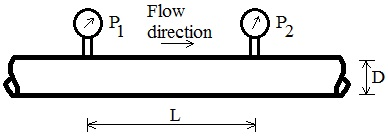Pressure Loss Equations

For steady flow of an incompressible fluid in a constant diameter horizontal pipe using the Darcy-Weisbach friction loss equation, the energy equation for flow from location 1 to 2 is expressed in terms of pressure loss as: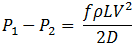where: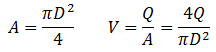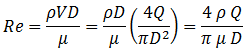For pressure loss, the Blasius equation represents the Moody friction factor for smooth pipes and 4000 ≤ Re ≤ 105 (Streeter et al., 1998, p. 291):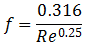Pressure loss unit abbreviations, symbols: kg=kilogram, m=meter, N=Newton, s=second. A=Pipe area.

Reference for pressure loss: Streeter, V. L., Wylie, E. B, and Bedford, K. W. 1998. Fluid Mechanics. WCB/McGraw-Hill. 3ed.